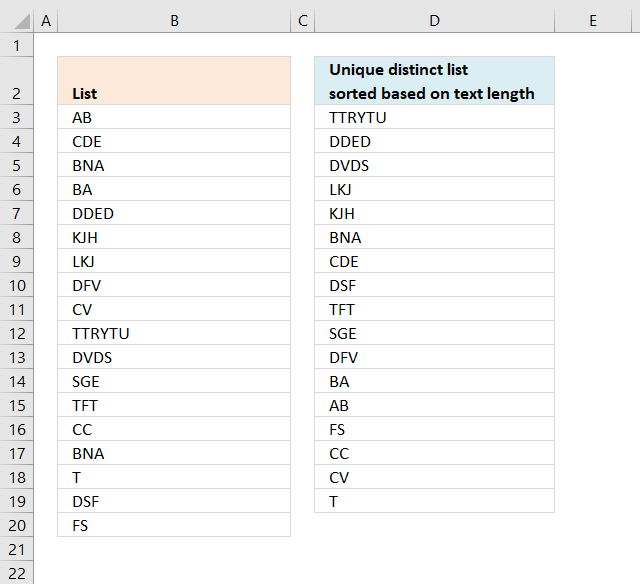Author: Oscar Cronquist Article last updated on December 04, 2018The formula in cell D3 extracts unique distinct values from B3:B20 sorted based on the number of characters, it works only with values in one column meaning your source range can't contain multiple columns.

Array formula in D3:

=IF(SUM(NOT(COUNTIF(\$D\$2:D2, \$B\$3:\$B\$20))*1)=0, "", INDEX(\$B\$3:\$B\$20, MATCH(MAX(LEN(\$B\$3:\$B\$20)*NOT(COUNTIF(\$D\$2:D2, \$B\$3:\$B\$20))), LEN(\$B\$3:\$B\$20)*NOT(COUNTIF(\$D\$2:D2, \$B\$3:\$B\$20)), 0)))

To enter an array formula, type the formula in a cell then press and hold CTRL + SHIFT simultaneously, now press Enter once. Release all keys.

The formula bar now shows the formula with a beginning and ending curly bracket telling you that you entered the formula successfully. Don't enter the curly brackets yourself.

### Explaining formula in cell D3

Step 1 and 2 makes sure that blanks are returned when all values have been shown.

#### Step 1 - Count unique distinct values

The formula returns blank values when all unique distinct values have been returned, the COUNTIF function lets you count each prior value against the list in cell range B3:B20.

COUNTIF(\$D\$2:D2,\$B\$3:\$B\$20)

becomes

COUNTIF("Unique distinct list sorted based on text length",{"AB"; "CDE"; "BNA"; "BA"; "DDED"; "KJH"; "LKJ"; "DFV"; "CV"; "TTRYTU"; "DVDS"; "SGE"; "TFT"; "CC"; "BNA"; "T"; "DSF"; "FS"})

and returns the following array:

{0;0;0;0;0;0;0;0;0;0;0;0;0;0;0;0;0;0}

The array tells us that no value has been shown yet.

The NOT function converts TRUE to FALSE or vice versa and 1 to 0 and 0 to 1.

NOT(COUNTIF("Unique distinct list sorted based on text length",{"AB"; "CDE"; "BNA"; "BA"; "DDED"; "KJH"; "LKJ"; "DFV"; "CV"; "TTRYTU"; "DVDS"; "SGE"; "TFT"; "CC"; "BNA"; "T"; "DSF"; "FS"}))

becomes

NOT({0;0;0;0;0;0;0;0;0;0;0;0;0;0;0;0;0;0})

and returns {TRUE; TRUE; TRUE; TRUE; TRUE; TRUE; TRUE; TRUE; TRUE; TRUE; TRUE; TRUE; TRUE; TRUE; TRUE; TRUE; TRUE; TRUE}.

Then we multiply with 1 to convert the boolean values into the corresponding numerical value. TRUE -> 1 and FALSE -> 0.

{TRUE; TRUE; TRUE; TRUE; TRUE; TRUE; TRUE; TRUE; TRUE; TRUE; TRUE; TRUE; TRUE; TRUE; TRUE; TRUE; TRUE; TRUE}*1 returns {1;1;1;1;1;1;1;1;1;1;1;1;1;1;1;1;1;1}.

The SUM function adds each value in the array .

SUM({1;1;1;1;1;1;1;1;1;1;1;1;1;1;1;1;1;1})

and returns 18.

#### Step 2 - Compare the number to 0 (zero)

When we copy the formula and paste to cells below the cell reference expands and counts prior values. The IF function lets you decide what will happen when the logical expression returns TRUE and FALSE.

IF(SUM(NOT(COUNTIF(\$D\$2:D2, \$B\$3:\$B\$20))*1)=0, "", formula)

becomes

IF(18=0, "", formula)

becomes

IF(FALSE, "", formula)

and returns the formula. The following steps explains the formula.

#### Step 3 - Count characters

The LEN function counts the number of characters in one cell, this is an array formula so the LEN function calculates the length of each value in B3:B20 and returns an array of length values.

LEN(\$B\$3:\$B\$20)

returns

{2; 3; 3; 2; 4; 3; 3; 3; 2; 6; 4; 3; 3; 2; 3; 1; 3; 2}

#### Step 4 - Check previous values

The COUNTIF function counts values based on a condition or criteria. In this case the COUNTIF function counts previous values in order to prevent duplicate values from showing up.

COUNTIF(\$D\$2:D2, \$B\$3:\$B\$20)

returns

{0;0;0;0;0;0;0;0;0;0;0;0;0;0;0;0;0;0}

#### Step 5 - Convert FALSE to TRUE

The NOT function converts boolean values, TRUE (1) to FALSE (0) and FALSE (0) to TRUE (1).

NOT(COUNTIF(\$D\$2:D2, \$B\$3:\$B\$20))

becomes

NOT({0;0;0;0;0;0;0;0;0;0;0;0;0;0;0;0;0;0})

and returns {TRUE; TRUE; TRUE; TRUE; TRUE; TRUE; TRUE; TRUE; TRUE; TRUE; TRUE; TRUE; TRUE; TRUE; TRUE; TRUE; TRUE; TRUE}.

#### Step 6 - Multiply arrays

LEN(\$B\$3:\$B\$20)*NOT(COUNTIF(\$D\$2:D2, \$B\$3:\$B\$20))

becomes

{2; 3; 3; 2; 4; 3; 3; 3; 2; 6; 4; 3; 3; 2; 3; 1; 3; 2}* {TRUE; TRUE; TRUE; TRUE; TRUE; TRUE; TRUE; TRUE; TRUE; TRUE; TRUE; TRUE; TRUE; TRUE; TRUE; TRUE; TRUE; TRUE}

and returns

{2;3;3;2;4;3;3;3;2;6;4;3;3;2;3;1;3;2}.

#### Step 7 - Find largest value in array

The MAX function returns the largest number in the array.

MAX(LEN(\$B\$3:\$B\$20)*NOT(COUNTIF(\$D\$2:D2, \$B\$3:\$B\$20)))

becomes

MAX({2;3;3;2;4;3;3;3;2;6;4;3;3;2;3;1;3;2})

and returns 6.

#### Step 8 - Match the largest value to find the relative position in the array

MATCH(MAX(LEN(\$B\$3:\$B\$20)*NOT(COUNTIF(\$D\$2:D2, \$B\$3:\$B\$20))), LEN(\$B\$3:\$B\$20)*NOT(COUNTIF(\$D\$2:D2, \$B\$3:\$B\$20)), 0)

becomes

MATCH(6, LEN(\$B\$3:\$B\$20)*NOT(COUNTIF(\$D\$2:D2, \$B\$3:\$B\$20)), 0)

becomes

MAX(6, {2;3;3;2;4;3;3;3;2;6;4;3;3;2;3;1;3;2}, 0)

and returns 10.

#### Step 9 - Return the value from position 10.

INDEX(\$B\$3:\$B\$20, MATCH(MAX(LEN(\$B\$3:\$B\$20)*NOT(COUNTIF(\$D\$2:D2, \$B\$3:\$B\$20))), LEN(\$B\$3:\$B\$20)*NOT(COUNTIF(\$D\$2:D2, \$B\$3:\$B\$20)), 0))

becomes

INDEX(\$B\$3:\$B\$20, 10)

and returns TTRYTU in cell D3.